## Backward Difference

The backward difference is a Finite Difference defined by(1)

Higher order differences are obtained by repeated operations of the backward difference operator, so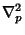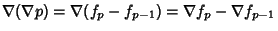(2)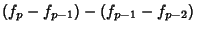(3)

In general,(4)

where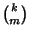is a Binomial Coefficient.

Newton's Backward Difference Formula expressesas the sum of theth backward differences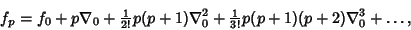(5)

where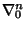is the firstth difference computed from the difference table.

See also Adams' Method, Difference Equation, Divided Difference, Finite Difference, Forward Difference, Newton's Backward Difference Formula, Reciprocal Difference

References

Beyer, W. H. CRC Standard Mathematical Tables, 28th ed. Boca Raton, FL: CRC Press, pp. 429 and 433, 1987.